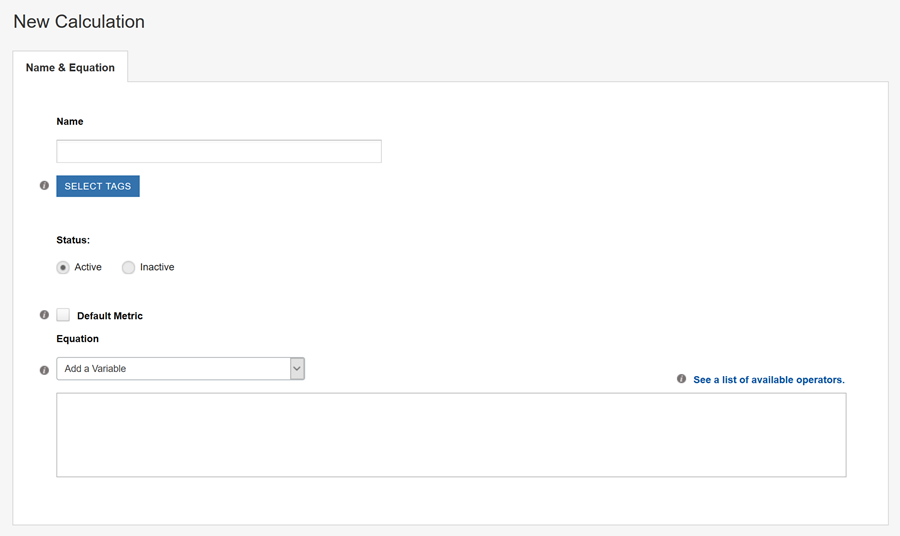Help Center

# Defining a Calculation

To open the Calculations page, select New and then Calculation.The top of the page contains basic information: NameTags, and Status.

Default Metric – Selects the Metric by default when you create a new Campaign.

Equation – At the bottom of the page, the Equation field allows you to build the Calculation using a combination of SiteSpect Variables and various operators.

To build a Calculation in the Equation field:

1. Select an item from the Add a Variable drop-down.
2. Add any of the available operators. The operators are listed in the tooltip for the Equation field as well as the Calculation Operators page.
3. Select another item from the Add a Variable list.
4. Repeat these steps until you've built your Equation.

To add a variable to the Equation, select it from the Add a Variable list.

To delete a variable from the Equation, click the small x that appears at the end of the variable.